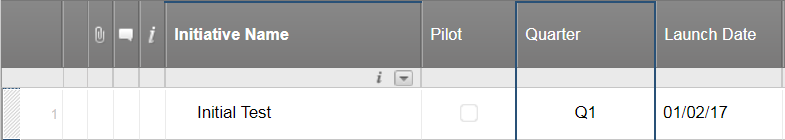#### Welcome to the Smartsheet Forum Archives

The posts in this forum are no longer monitored for accuracy and their content may no longer be current. If there's a discussion here that interests you and you'd like to find (or create) a more current version, please Visit the Current Forums.

# Formula For Finding Quarter

Hello,

New to smartsheets I have been using excel extensively.  Can someone pleased teach me the formula for finding a quarter based on a date?

For instance [Date Data Column]1 = 1/1/2016 I want the formula to return "Q1" in the [Quarter]1 cell

Havent been able to figure this one out...help please!

Tags:
«1

• Hi there Cat-- We don't offer a built-in expression to determine a quarter based on a date, but I'll add your vote for this function to our enhancement request list. Thanks for your input!

In the meantime, you could build an nested IF statement to determine the quarter manually. It'll look similar to the example below:

=IF(ISDATE([Date]13), IF(MONTH([Date]13) = 1, "Q1", IF(MONTH([Date]13) = 2, "Q1", IF(MONTH([Date]13) = 3, "Q1", IF(MONTH([Date]13) = 4, "Q2", IF(MONTH([Date]13) = 5, "Q2", IF(MONTH([Date]13) = 6, "Q2", IF(MONTH([Date]13) = 7, "Q3", IF(MONTH([Date]13) = 8, "Q3", IF(MONTH([Date]13) = 9, "Q3", IF(MONTH([Date]13) = 10, "Q4", IF(MONTH([Date]13) = 11, "Q4", IF(MONTH([Date]13) = 12, "Q4")))))))))))))

You'll want to modify the "[Date]13" reference to match the first date cell in your sheet that you want to determine the quarter of. Then, you can drag-fill the formula down any existing rows. Let me know how this works for you.

• This is a little cleaner and uses less computer resources

=IF(ISDATE(Date14), IF(MONTH(Date14) <= 3, "Q1", IF(MONTH(Date14) <= 6, "Q2", IF(MONTH(Date14) <= 9, "Q3", "Q4"))))

Craig

• Craig-- Good catch! I'll add that to my formula reference sheet.

• Could I put a vote in for adding a function for Quarter like the one that Excel has? We use Quarters a lot in reporting and this would make life much easier than including formulas that are as complicated as the one above.

• Hello,

I have the same question, but I would like it to return a year ("Q1 - 2017" or "Q1 - 2018"). Something similar to the following Excel function if possible:

="Q" &INT((MONTH(A1)+2)/3) & "-" & YEAR(A1)

Thank you for the help!• Disregard. I found a way to make this work through using a hidden "Year" column containing =YEAR([Launch Date]1) and using the following function in the Quarter column:

=IF(ISDATE([Launch Date]1), IF(MONTH([Launch Date]1) <= 3, "Q1-" + [Year]1, IF(MONTH([Launch Date]1) <= 6, "Q2-" + [Year]1, IF(MONTH([Launch Date]1) <= 9, "Q3-" + [Year]1, "Q4-" + [Year]1))))

Is there a better option without using an additional column?

• Hi Cat,

Here are two cleaner formulas for determining Quarter.

This will return "Q#":

="Q" + INT((MONTH([Column 1]1) + 2) / 3)

This will return "Q# - YYYY":

="Q" + INT((MONTH([Column 1]1) + 2) / 3) + " - " + YEAR([Column 1]1)

• Disregard.  I have worked out a solution.

Hi, I am using the formula you provided for parsing a quarter.

=IF(ISDATE([Projected Go Live]52), IF(MONTH([Projected Go Live]52) <= 3, "Q1", IF(MONTH([Projected Go Live]52) <= 6, "Q2", IF(MONTH([Projected Go Live]52) <= 9, "Q3", IF(MONTH([Projected Go Live]52) <= 12, "Q4")))))

My issue is cell [Projected Go Live]52 has a value "TBD" for projects with an undetermined date.  I would like TBD to display in the cell anytime this value appears.

How would I attempt this?

Thank you.

• Craig

how do I add the year ?

in xls I use =RIGHT(YEAR(date field),4)&" - "&"Q"&INT((MONTH(date field)/4)+1)

but this does not work in smartsheet.

Géraldine

• Smartsheet uses "+" not "&" for concatenation.

For the [Due Date] column, row 23:

If you want the full year (like your formula shows), then you don't need RIGHT()

=YEAR([Due Date]23) + " - " + "Q" + INT((MONTH([Due Date]23) / 4) + 1)

If you want only the 2 digit year, then you do (but use RIGHT(YEAR(),2) instead.

=RIGHT(YEAR([Due Date]23), 2) + " - " + "Q" + INT((MONTH([Due Date]23) / 4) + 1)

Craig

• Craig

I choose the first option. thanks a lot!

• Hi! I'm looking to have a formula that adds the Quarter - Year. Except that my quarter starts October 1.

I saw the simplified formula which is great but was wondering if anyone knew how to customize to relevant fiscal year.

• Hi Renee - I am new to smart sheets and realize your comment is more than a year old, but i didn't see a response. I think you will need to nest one more "if" statment and it would like something like IF([Projected Go Live]52 = TBD, "TBD")

The main thing I am unsure about is if it should be = TBD or = "TBD", but I think that should be a quick test. I think you should be able to add that nested field in at the end so your new formula would look something like:

=IF(ISDATE([Projected Go Live]52), IF(MONTH([Projected Go Live]52) <= 3, "Q1", IF(MONTH([Projected Go Live]52) <= 6, "Q2", IF(MONTH([Projected Go Live]52) <= 9, "Q3", IF(MONTH([Projected Go Live]52) <= 12, "Q4",IF([Projected Go Live]52 = “TBD”, “TBD”))))))

• Same question here. Anyone can help please?

• Is there a way to calculate the first day of quarter end date based on today's day? i.e. for today, I would want a cell to populate 10/1/2019.

This discussion has been closed.